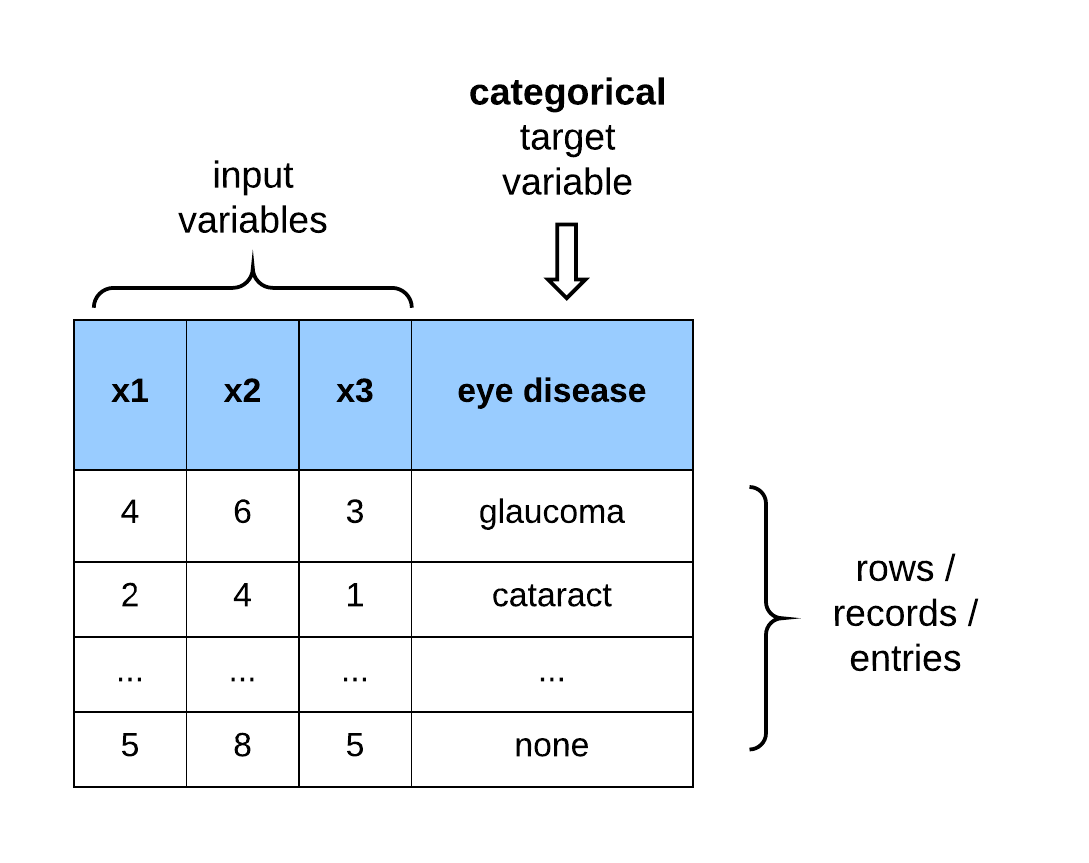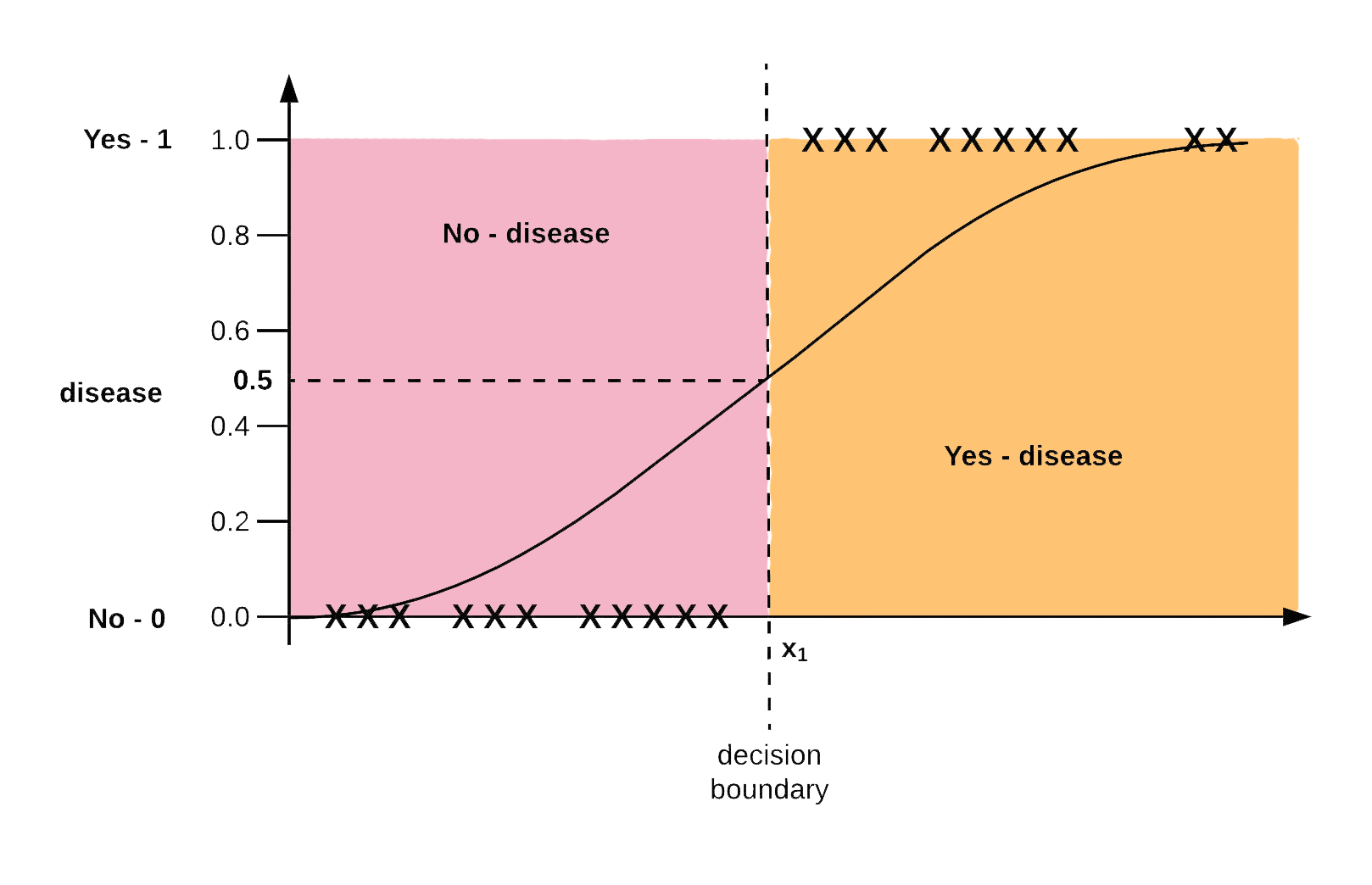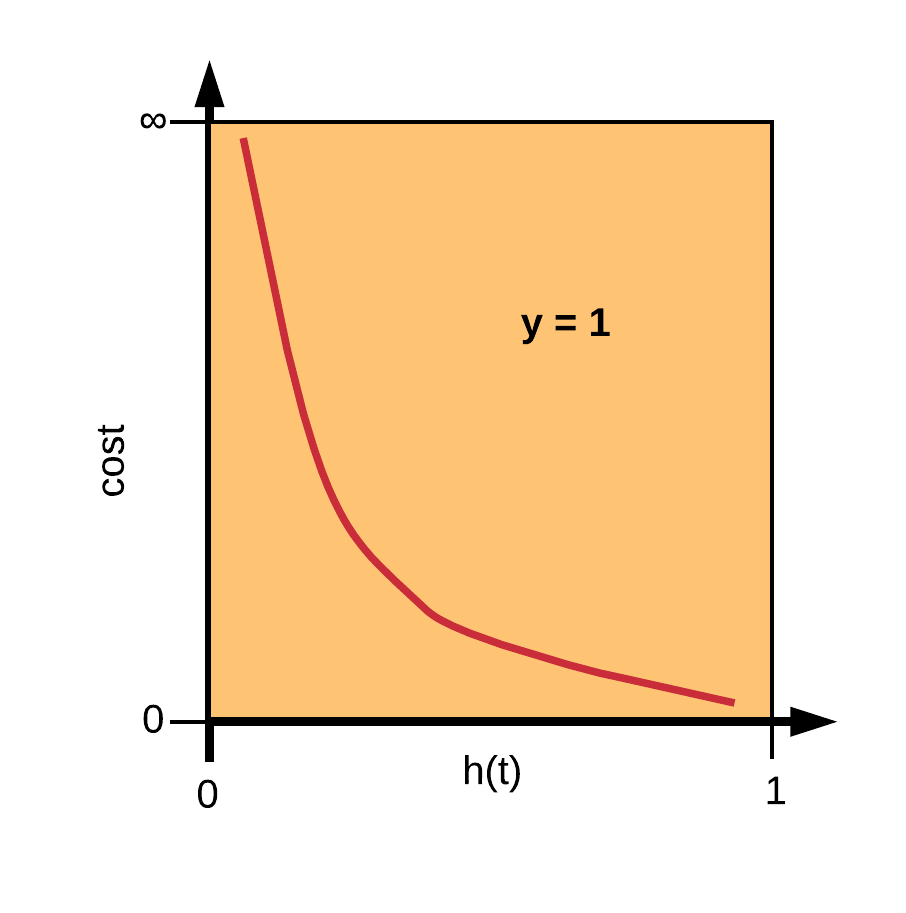Logistic regression is a supervised machine learning algorithm developed for learning classification problems.## The Logit or Sigmoid Model

The logistic function, also known as logit or sigmoid function constrains the output of the cost function as a probability between 0 and 1. The sigmoid function is formally written as:

Logistic regression is also parametric as shown below:

where, $0\leq h(t)\leq1.$ The sigmoid function is illustrated below:The sigmoid function, resembing an $S$ curve, rises from 0 and plateaus at 1. As $X_1$ increases to infinity the sigmoid output gets closer to 1, and as $X_1$ decreases towards negative infinity, the sigmoid function outputs 0.

## Logistic Regression Cost Function

The logistic regression cost function is formally written as:

The cost function also known as log-loss, is set up in this form to output the penalty made on the algorithm if $h(t)$ predicts one class, and the actual output is another.## Logistic Regression Model with TensorFlow Canned Estimators

# import packages
import numpy as np
import tensorflow as tf
from sklearn import datasets
from sklearn.model_selection import train_test_split
from sklearn.preprocessing import OneHotEncoder

# load dataset

# separate features and target
X = data.data
y = data.target

# split in train and test sets
X_train, X_test, y_train, y_test = train_test_split(X, y, shuffle=True)


### Tensorflow Datasets (tf.data)

The Datasets package (tf.data) provides a convenient set of high-level functions for creating complex dataset input pipelines. The goal of the Dataset package is to have a fast, flexible and easy to use interface for fetching data from various data sources, performing data transform operations on them before passing them as inputs to the learning model.

# create an input_fn
def input_fn(X, y, batch_size=30, training=True):
# convert to dictionary
X = {'sepal_length': X[:,0],
'sepal_width':  X[:,1],
'petal_length': X[:,2],
'petal_width':  X[:,3]}
dataset = tf.data.Dataset.from_tensor_slices((X, y))
if training:
dataset = dataset.shuffle(buffer_size=1000)
dataset = dataset.repeat()
dataset = dataset.batch(batch_size)
iterator = dataset.make_one_shot_iterator()
features, labels = iterator.get_next()
return features, labels


### FeatureColumns

TensorFlow offers a high-level API called FeatureColumns tf.feature_column for describing the features of the dataset that will be fed into an Estimator for training and validation. This makes easy the preparation of data for modeling, such as the conversion of categorical features of the dataset into a one-hot encoded vector.

# use feature columns to define the attributes to the model
sepal_length = tf.feature_column.numeric_column('sepal_length')
sepal_width = tf.feature_column.numeric_column('sepal_width')
petal_length = tf.feature_column.numeric_column('petal_length')
petal_width = tf.feature_column.numeric_column('petal_width')
feature_columns = [sepal_length, sepal_width, petal_length, petal_width]


### Estimators

The Estimator API is a high-level TensorFlow functionality that is aimed at reducing the complexity involved in building machine learning models by exposing methods that abstract common models and processes. There are two ways of working with Estimators and they include:

• Using the Pre-made Estimators: The pre-made Estimators, are black-box models for building common machine learning and deep learning architectures such as Linear Regression/ Classification, Random Forests Regression/ Classification and Deep Neural Networks for regression and classification.
• Creating a Custom Estimator: It is also possible to use the low-level TensorFlow methods to create a custom black-box model for easy reusability.
# instantiate a DNNLinearCombinedClassifier Estimator
estimator = tf.estimator.DNNLinearCombinedClassifier(
dnn_feature_columns=feature_columns,
dnn_hidden_units=,
dnn_activation_fn=tf.nn.relu,
n_classes=3
)

INFO:tensorflow:Using default config.
WARNING:tensorflow:Using temporary folder as model directory: /var/folders/gh/mqsbbqy55bb4z763xxgddw780000gn/T/tmpicufr3mp
INFO:tensorflow:Using config: {'_is_chief': True, '_task_id': 0, '_tf_random_seed': None, '_session_config': allow_soft_placement: true
graph_options {
rewrite_options {
meta_optimizer_iterations: ONE
}
}
, '_save_checkpoints_steps': None, '_evaluation_master': '', '_cluster_spec': <tensorflow.python.training.server_lib.ClusterSpec object at 0x12b4cbf98>, '_keep_checkpoint_every_n_hours': 10000, '_train_distribute': None, '_save_summary_steps': 100, '_device_fn': None, '_keep_checkpoint_max': 5, '_num_ps_replicas': 0, '_global_id_in_cluster': 0, '_log_step_count_steps': 100, '_experimental_distribute': None, '_save_checkpoints_secs': 600, '_service': None, '_model_dir': '/var/folders/gh/mqsbbqy55bb4z763xxgddw780000gn/T/tmpicufr3mp', '_eval_distribute': None, '_master': '', '_num_worker_replicas': 1, '_task_type': 'worker', '_protocol': None}

# train model
estimator.train(input_fn=lambda:input_fn(X_train, y_train), steps=2000)

INFO:tensorflow:Calling model_fn.
INFO:tensorflow:Done calling model_fn.
INFO:tensorflow:Create CheckpointSaverHook.
INFO:tensorflow:Graph was finalized.
INFO:tensorflow:Running local_init_op.
INFO:tensorflow:Done running local_init_op.
INFO:tensorflow:Saving checkpoints for 0 into /var/folders/gh/mqsbbqy55bb4z763xxgddw780000gn/T/tmpicufr3mp/model.ckpt.
INFO:tensorflow:loss = 110.14471, step = 1
INFO:tensorflow:global_step/sec: 580.339
INFO:tensorflow:loss = 31.621853, step = 101 (0.173 sec)
INFO:tensorflow:global_step/sec: 905.215
INFO:tensorflow:loss = 18.581322, step = 201 (0.111 sec)
INFO:tensorflow:global_step/sec: 905.436
INFO:tensorflow:loss = 13.926304, step = 301 (0.110 sec)
INFO:tensorflow:global_step/sec: 888.803
INFO:tensorflow:loss = 15.127607, step = 401 (0.113 sec)
INFO:tensorflow:global_step/sec: 889.838
INFO:tensorflow:loss = 9.994065, step = 501 (0.112 sec)
INFO:tensorflow:global_step/sec: 970.035
INFO:tensorflow:loss = 10.063839, step = 601 (0.102 sec)
INFO:tensorflow:global_step/sec: 895.97
INFO:tensorflow:loss = 7.3032517, step = 701 (0.112 sec)
INFO:tensorflow:global_step/sec: 923.735
INFO:tensorflow:loss = 7.5960145, step = 801 (0.107 sec)
INFO:tensorflow:global_step/sec: 897.826
INFO:tensorflow:loss = 6.296457, step = 901 (0.111 sec)
INFO:tensorflow:global_step/sec: 782.357
INFO:tensorflow:loss = 6.184272, step = 1001 (0.128 sec)
INFO:tensorflow:global_step/sec: 906.255
INFO:tensorflow:loss = 4.6410484, step = 1101 (0.111 sec)
INFO:tensorflow:global_step/sec: 930.795
INFO:tensorflow:loss = 4.5383587, step = 1201 (0.107 sec)
INFO:tensorflow:global_step/sec: 964.033
INFO:tensorflow:loss = 4.7723937, step = 1301 (0.104 sec)
INFO:tensorflow:global_step/sec: 889.823
INFO:tensorflow:loss = 2.7989619, step = 1401 (0.112 sec)
INFO:tensorflow:global_step/sec: 948.613
INFO:tensorflow:loss = 3.7111075, step = 1501 (0.105 sec)
INFO:tensorflow:global_step/sec: 905.985
INFO:tensorflow:loss = 4.1749625, step = 1601 (0.110 sec)
INFO:tensorflow:global_step/sec: 938.932
INFO:tensorflow:loss = 3.5651736, step = 1701 (0.106 sec)
INFO:tensorflow:global_step/sec: 901.104
INFO:tensorflow:loss = 4.0226192, step = 1801 (0.111 sec)
INFO:tensorflow:global_step/sec: 836.779
INFO:tensorflow:loss = 1.3753442, step = 1901 (0.119 sec)
INFO:tensorflow:Saving checkpoints for 2000 into /var/folders/gh/mqsbbqy55bb4z763xxgddw780000gn/T/tmpicufr3mp/model.ckpt.
INFO:tensorflow:Loss for final step: 0.95693886.

<tensorflow.python.estimator.canned.dnn_linear_combined.DNNLinearCombinedClassifier at 0x12b4cbf28>

# evaluate model
metrics = estimator.evaluate(input_fn=lambda:input_fn(X_test, y_test, training=False))

INFO:tensorflow:Calling model_fn.
INFO:tensorflow:Done calling model_fn.
INFO:tensorflow:Starting evaluation at 2019-02-01-02:40:26
INFO:tensorflow:Graph was finalized.
INFO:tensorflow:Restoring parameters from /var/folders/gh/mqsbbqy55bb4z763xxgddw780000gn/T/tmpicufr3mp/model.ckpt-2000
INFO:tensorflow:Running local_init_op.
INFO:tensorflow:Done running local_init_op.
INFO:tensorflow:Finished evaluation at 2019-02-01-02:40:26
INFO:tensorflow:Saving dict for global step 2000: accuracy = 0.9736842, average_loss = 0.104831874, global_step = 2000, loss = 1.9918057
INFO:tensorflow:Saving 'checkpoint_path' summary for global step 2000: /var/folders/gh/mqsbbqy55bb4z763xxgddw780000gn/T/tmpicufr3mp/model.ckpt-2000

print('\nTest set accuracy: {accuracy:0.3f}\n'.format(**metrics))

Test set accuracy: 0.974